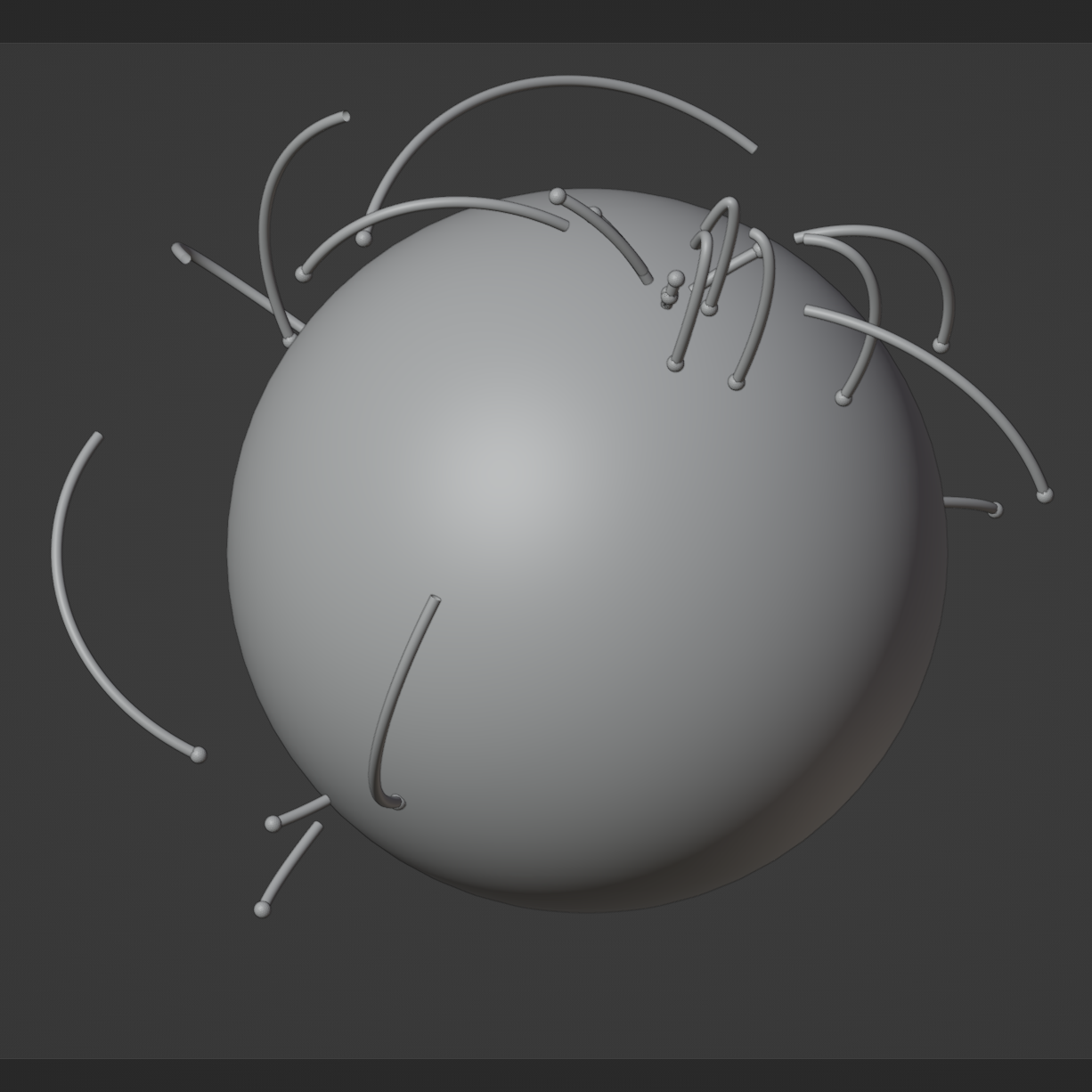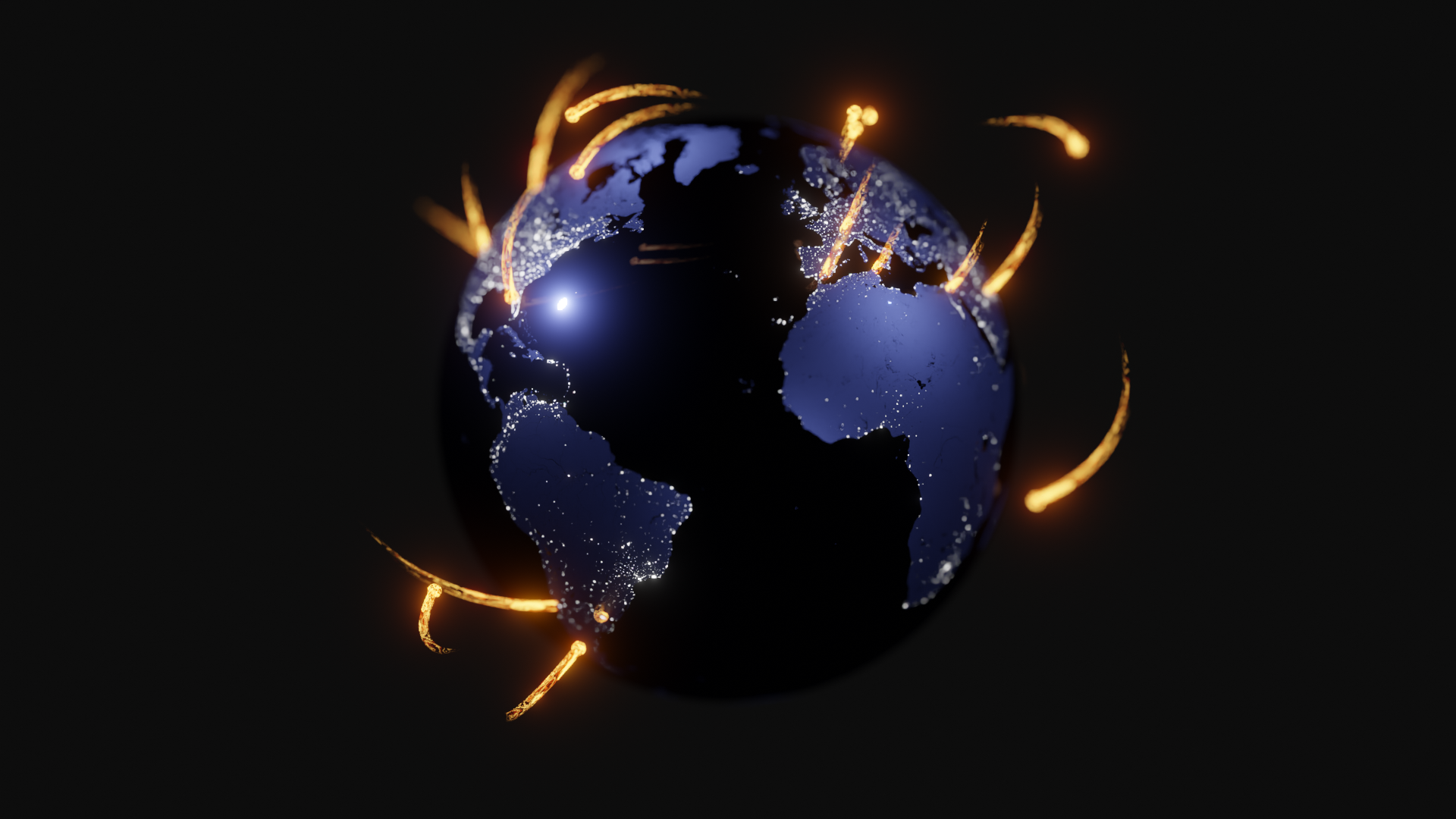# Globe Flights

Created as part of #30DayMapChallenge

Behind the Scenes

In this ‘Behind the Scenes’, I’ll cover drawing an arc in 3D. For the final animation, I built on these basic arc paths with objects moving on this path and creating a fire trail along the path. These two techniques will be covered in Space and Shipping Movements posts…

How hard can it be to draw an arc? Blender has a sphere object under ‘Add->Mesh->Ico Sphere’ and a point on the surface of the sphere has co-ordinates (x,y,z) defined by:

``````
# R is the radius of the sphere. The earth's radius is 6,400km but we can scale this down to R=6.4 within Blender
def getXYZfromLatLonVector(R, lat, lon):
# the x,y radius changes with lat
x = r * math.sin(math.radians(lon)) * -1
return mathutils.Vector((x,y,z))
``````

If we have two points defined by their latitudes and longitudes and a formula to create cartesian co-ordinates, can’t we just interpolate? Not quite it seems. I spent quite a while reading up on spherical co-ordinates, searching the web and reading math.stackexchange.com but, after implementing, none of the arcs looked quite right. I can’t remember how I ended up on the Blender documentation page, but I did and there’s a curiously named function slerp()

Function Description
slerp(other, factor) Returns the interpolation of two quaternions

Seems it’s exactly what we need!

``````def testBlenderSlerp():
R = 6.4
# London
lat = 51.5072
lon = -0.1275
AVector = getXYZfromLatLonVector(R, lat, lon)
print(f"AVector: {AVector}")

# New York
lat = 40.7834
lon =  -73.9662
BVector = getXYZfromLatLonVector(R, lat, lon)
print(f"BVector: {BVector}")

points = []
for step in range (-1, 12, 1):
increment = step / 10
newPoint = AVector.copy()
slerp = AVector.slerp(BVector, increment)
newPoint = scaleVector(slerp, getScale(R, increment))
points.append(newPoint)